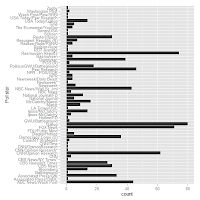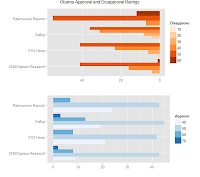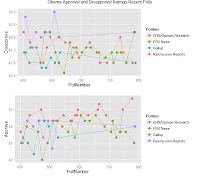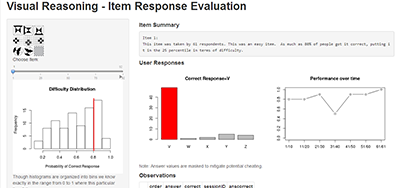## Thursday, May 30, 2013

### Are Fox News Polls Biased?

Especially after the outcome of the mid-term election, I think there is a common contention among some groups that there is something wonky about Fox News when it comes to reporting polls relative to President Obama and the Democratic Party in general.Graph 1
Looking at the 795 polls (RealClearPolitics.com) thus far produced shows that there are 55 different pollsters, with the top 4 are Fox, CNN, Gallup, and Rasmussen (See Graph 1).  Taking a more detailed look at the top 4 we see that there seems to be a much larger proportional block of those who disapprove of the president in the Rasmussen Reports and Fox News relative to Gallup and CNN (Graph 2).  A similar pattern appears in the approval polls numbers.

Over time Obama's ratings have moved quite a bit.  It is hard to identify a clear pattern as far as one pollster being higher than another in the long run (Graph 3) though in the short run (Graph 4) it appears that CNN consistently reports high approval numbers and Rasmussen consistently reports low approval values.Graph 2
When comparing polls among the top 4 there is no sense of objectiveness since there is no clarity as to which poll is closer to the objective truth if there is systematic bias in one or more of the pollsters.  However, what we can instead do is compare the polls to that of the average within each poll collection phase (say every 50 polls).  Removing the time average for every 50 polls we can see a bit more clearly how each pollster is behaving (Graph 5)

A pollster will be above the average 50% of the time and below the average 50% of the time if the pollster is unbiased and the average is unbiased.   We can see that for the polls looking at the average approval gap (Table 1, Column 3 and 4). In a similar manner by looking at how individual polls diverge from the average poll, we can take the average of this divergence.  If the poll is fair then the average gap will be close to zero.  If this gap is positive then it means on average the pollster reports polls levels of either approval or disapproval above the average poll value for the period.  If the average is negative then it means the pollster reported average is less than the polling average (Table 1, Column 1 and 2).
Looking at Table 1 we can see that CNN tends to over-report both approval and disapproval numbers relative to the typical poll.  CNN is more likely to over-report disapproval numbers.  Fox on the other hand
is prove to under-reporting both approval and disapproval numbers though Fox is much more likely to under-report approval numbers.  Gallup on the other hand is almost the mirror of Fox except that it tends to much more heavily under-report disapproval numbers rather than approval numbers.Graph 4
Finally, looking at the Rasmussen Reports it is clear that there is something fishy going on.  The average disapproval rating report is nearly 7 points above those of other polling locations while the approval rating is under-reported by nearly 2 points.  In addition, every poll that is put out by Rasmussen has reported 100% of the times disapproval rates above the average.  This is an astounding number since it is clearly highly unlikely.

However, since I am not an expert in polling at all, I am not sure if Rasmussen is doing something legitimate and well known.  It is very clear to me from the data however that they are not to be considered equivalent to the other three major polling agencies.  Looking at wikipedia, it is well understood that Rasmussen has a different type of polling by selecting individual with a plan to only capture "likely voters".
Overall it looks like Fox is off the hook.  Table 2 shows them slightly under-reporting approval rates while CNN is over-reporting.  The difference in disapproval rates though is not statistically significant for Fox though it is for CNN as well (Table 3)

Find the code to produce all of these results at:
https://github.com/EconometricsBySimulation/2013-05-30-FoxNews/blob/master/analysis.r

Table 1: Averages and counts
 Average Gap Count Approve Disapprove Approve Disapprove CNN/Opinion Research 1.23 1.90 0.63 0.69 FOX News -1.40 -0.31 0.31 0.44 Gallup -0.41 -1.53 0.38 0.34 Rasmussen Reports -1.86 6.73 0.32 1.00

Table 2: T-tests of Approval Gap
 Estimate Std. Error t value Pr(>|t|) PollsterCNN/Opinion Research 1.232823 0.471684 2.613664 0.009437 PollsterFOX News -1.40239 0.440775 -3.18165 0.001627 PollsterGallup -0.41156 0.415242 -0.99114 0.322465 PollsterRasmussen Reports -1.85953 0.431748 -4.30697 2.28E-05

Table 3: T-tests of Disapproval Gap
 Estimate Std. Error t value Pr(>|t|) PollsterCNN/Opinion Research 1.896855 0.572502 3.313273 0.001042 PollsterFOX News -0.31254 0.534987 -0.58419 0.559557 PollsterGallup -1.52794 0.503997 -3.03164 0.002658 PollsterRasmussen Reports 6.7325 0.524031 12.84753 4.51E-30

## Wednesday, May 29, 2013

### Item Analysis App - Shiny Code

```Here is the code for my first Shiny App! It is the one that I posted previously with a few slight revisions. You can see it at:A Shiny R App

In order to make any sense of this I suggest you working through the tutorial (http://www.rstudio.com/shiny/) it would be also useful to apply for beta access to the rstudio server which provides free hosting for experimental shiny applications.

To make the code below work you would need two files server.R and ui.R.  Read the tutorial and you will understand their importance.  After reading the tutorial and playing around a little I was able to create the following app.  Hope you can do the same!`````` Visual Reasoning Test v0.2b ```

`Please post as comments links to apps you have developed as well.  Also, feel free to use the data as needed.  It should continue to be updated as more people keep taking the test.`

`Francis`

`Code can be found at:`

`https://github.com/EconometricsBySimulation/2013-05-29-ShinyApp.git`

```Please tell me if I am doing this github thing wrong.  Should I have a different repo every post?

```
```# server.R
library(shiny)

# For this app I will load in data form the visual reasoning test that I posted to this blog last week (Visual Reasoning Test Link).```
` `
`# We have had a great deal of responses so the data is getting pretty rich!  Thanks so much :)`

```# I will come back to this generously generated data later!

# Load the item response data into memory with this somewhat odd formation.
con = url("http://concerto4.e-psychometrics.com/media/13/Visual.Reasoning1.RData")
close(con)
nrow(individual.responses)

# I specify input\$obs initially for debugging purposes.  Once this loads up on the server it is overwritten by the GUI.
input = list(obs=27)

# Make a vector of values to identify the session ID for each test taker
respondents = unique(individual.responses\$sessionID)

### Item Analysis

# Create a vector of item names
items = unique(individual.responses\$item)

# Calculate some values that will be useful later
responses.mean  = tapply(individual.responses\$anscorrect, individual.responses\$item, mean)
responses.count = tapply(individual.responses\$anscorrect, individual.responses\$item, length)

sum.responses = data.frame(items, mean=responses.mean, count=responses.count)

# hist(sum.responses\$mean, breaks=12, col=grey(.4), main="Histogram of Item Difficulties", xlab="Probability of Correct Response")

# This function takes the min of a vector and that of a scalar or two vectors of equal length.
tmin = function(v1,v2) {
r = NULL
if (length(v2)==1) v2=rep(v2,length(v1))
for (i in 1:length(v1)) r[i] = min(v1[i],v2[i])
return(r)
}
# A couple of examples
tmin(1:10,5)
tmin(1:10,10:1)

# END SERVER STARTUP

# Define server logic required to summarize and view the selected dataset
shinyServer(function(input, output) {

# Generate a summary of the item
output\$summary <- renderPrint({

# I want to calculate what percent of the item responses got it right and out of all responses how that compared with other items.
correct.mean = round(mean(responses.mean[input\$obs]),2)
percentile = round(mean(responses.mean<correct.mean),2)

dataset = individual.responses[individual.responses\$item==input\$obs,]

# I will save a number of text bits to combine together to a single text summary of the item.
text0 = paste0("Item ", input\$obs, ":\n")

text.5 = paste0("This item was taken by ", responses.count[input\$obs], " respondents. ")

text1 = "This was a very easy item.  As much as "
if ((percentile<.80)) text1 = "This was an easy item.  As much as "
if ((percentile<.60)) text1 = "This was an average item.  About "
if ((percentile<.40)) text1 = "This was a hard item.  Only "
if ((percentile<.20)) text1 = "This was a very hard item.  Only "

text2 = paste0(round(correct.mean,2)*100,"% of people got it correct, putting it in the ", 100-percentile*100, " percentile in terms of difficulty.")

text3 = ""
if ((loading > .5) & (correct.mean<.5)) text3 = paste0(" Note that there is a large loading on a response ", round(loading,2)*100 ,"% which is not the correct one. This probably indicates that there is something wrong with this problem.")

cat(paste0(text0,text.5,text1,text2, text3))
})

# Plot Item Difficulty
output\$distPlot <- renderPlot({
hist(responses.mean, xlab="Probability of Correct Response", main="Difficulty Distribution")
abline(v=responses.mean[input\$obs], lwd=3, col="red")
})

# Send item preview to the control bar
output\$preImage = renderImage({
# When input\$n is 3, filename is ./images/image3.jpeg
filename = normalizePath(file.path('Images', paste0('Q', input\$obs, '.png')))
# Return a list containing the filename and alt text
list(src = filename, alt = paste("Image number", input\$obs))
}, deleteFile = FALSE)

# Graph bar graph of responses
output\$respPlot <- renderPlot({
# Grab a subset of the item.response data to display
dataset = individual.responses[individual.responses\$item==input\$obs,]

# Set up the output for the plots that we would like.
par(mfrow=c(1,2))

# Select the color of the bar which if the right answer to be red.
barcol = c("grey", "grey", "grey", "grey", "grey")
barcol[sort(unique(individual.responses\$correct))==dataset\$correct]="red"
main=paste0("Correct Response=",dataset\$correct))

# Calculate the average number of correct responses per ten items.
avg.correct = tapply(dataset\$anscorrect, ceiling((1:length(dataset\$correct))/10), mean)
# Plot those respones over time.
plot(avg.correct, xaxt = "n", type="b", ylab="", xlab="",
main="Performance over time", ylim=c(0,1))
# Change the x axis to have custom tick labels.
navgs = length(avg.correct)
axis(1, at=1:length(avg.correct), paste0(1+(1:length(avg.correct)-1)*10,"/", (tmin((1:length(avg.correct))*10,length(dataset[])))))
})

# Show a table of all of the item response values.
output\$view = renderTable({
# Select the subset of data that pertains to the item selected.
dataset = individual.responses[individual.responses\$item==input\$obs,]
rownames(dataset) <- 1:nrow(dataset)
dataset\$ip <- dataset\$item <- dataset\$id <- NULL
dataset
}, digits=0)
})
```
```
```
```# ui.R
library(shiny)

# Define UI for dataset viewer application
shinyUI(pageWithSidebar(

# Application title.
headerPanel("Visual Reasoning - Item Response Evaluation"),

# This is the left hand panel.
sidebarPanel(
# This image is just loaded from another image and placed as a thumbnail into the shiny GUI.
imageOutput("preImage", width = "100px", height = "100px"),

# This allows the user to specify what the look of this input device will be.
# In this case a slider that has a min of 1 and max of 92.
sliderInput("obs", "Choose Item:",
min = 1, max = 92, value = 1, step= 1,
ticks=c(1,25,50,75,92) , animate=TRUE),

# This is the histogram of item difficulty
plotOutput("distPlot", height = "300px"),

# This displays text below the histogram
helpText("Though histograms are organized into bins we know exactly in the range from 0 to 1 where this particular item falls.")
),

# Now let's define the main panel.
mainPanel(
# Display the title.
h4("Item Summary"),
# Display the item summary table.
verbatimTextOutput("summary"),

h4("User Responses"),
# Display user response table.
plotOutput("respPlot", height = "300px"),
# Display the note.

h4("Observations"),
# Display the table output of item responses.
tableOutput("view"),

helpText("Order is the order that the item was given in in this particular user's experience.")
)
))```
```
```
Syntax Highlighting by Pretty R at inside-R.org

## Tuesday, May 28, 2013

### dapply - tapply for dataframes

```# Every so often I run into a situation when I find I need a function that seems to me that it should be very straightforward but because it seems so much so, it also becomes extremely difficult to find online.

# In this case, I am interested in a function that applies a function across and index of values and returns the results of that function as an equal length as the vector fed into the function.

# In the search for this specific function, I found the base function tapply(vector, index, function) which does very close to what I want.

# However, tapply returns a vector of length equal to the number of factors in the index value.

# From the tapply function there might be a very easy way to get what I want.

# The reason I want this function is for data analysis purposes.  Often times we might want to see how well a variable that changes on the individual level (such as student education) might affect a variable that changes on the district level (per pupil spending).

# However, nothing occured to me.  So I programmed up an alternative function which has a few more bells and whistles.  (Check out the comments below for efficient coding methods).```
```
# So hear it is.

dapply <- function(v1, index, fun, data=NULL, each=F) {
# If a data.frame or list is defined then grab v1 and index from them.
if (is.null(data)==F) {
# The deparse and substitute commands together
print(deparse(substitute(v1)))
v1 <- data[[deparse(substitute(v1))]]
index <- data[[deparse(substitute(index))]]
}
# Allow index to be a pattern in which case it is replicated accross the range of v1.
if (length(index)<length(v1)) {
if (length(index)*2>length(v1)) print(paste("Warning: Input vector is less than the index in length but not greater than twice."))
# Calculate the number of replications required to duplicate the length of v1 with the index.
ndup <- length(v1)/length(index)
# If the number is not a whole number warn user.
if (!is.integer(ndup)) print(gettextf("Input vector is of length %i can not be evenly divided by index of length %i.  Pattern truncated at end.", length(v1), length(index)))
# Repeat the index as neccessary
if (!each) index <- rep(index, times=ceiling(ndup))[1:length(v1)]
if (each)  index <- rep(index, each =ceiling(ndup))[1:length(v1)]
}

# Calculate the vector which will be returned.
vout <- tapply(v1, index, fun)

# Expand the vout to cover multiple returns
returner <- NULL
for (i in 1:length(index)) returner <- c(returner, vout[index[i]==names(vout)])
returner
}

# For the vector 1:100 we will find the sum of the each block of twenty numbers.
dapply(1:100, ceiling(1:100/20),sum)

# We could have instead specified the index from 1:5 and by specifying each=T it tells it to multiply each element by what is neccessary to equal the length of the input vector.
dapply(1:100, 1:5, sum, each=T)

# Without each we will instead have the sum of all of the every fifth number.
dapply(1:100, 1:5, sum)

# But more in line with the uses I am looking for let's first construct a sample data frame:
district <- ceiling(1:1000/100)
stud.achievement <- rnorm(1000)+district*.1
sampleframe <- data.frame(id=1:1000, stud.achievement, district)

# Now let's see if we can find average student achievment by district and save it as a new variable.
sampleframe\$Ave.ach <- dapply(stud.achievement, district, mean, data=sampleframe)

# Note, this function works very similarly to the egen command in Stata.```
Syntax Highlighted by Pretty R at inside-R.org

`# Gethub repository.`
https://github.com/EconometricsBySimulation/2013-05-28-dapply